# Change Font Size of ggplot2 Plot in R (5 Examples) | Axis Text, Main Title & Legend

In this article, I’ll explain how to increase and decrease the text font sizes of ggplot2 plots in R.

The tutorial consists of these content blocks:

Let’s do this:

## Example Data

In the examples of this R tutorial, I’ll use the following ggplot2 plot as basis. In order to create our example plot, we first need to create a data frame:

```data <- data.frame(Probability = c(0.5, 0.7, 0.4), # Example data Groups = c("Group A", "Group B", "Group C"))```

Our example data consists of two columns: A column containing some probability values and another column containing certain groups.

If we want to draw a ggplot2 plot of this data frame, we need to install and load the ggplot2 package to R:

```install.packages("ggplot2") # Install ggplot2 library("ggplot2") # Load ggplot2```

Now, we can draw a barchart of our data as follows:

```my_ggp <- ggplot(data, # Basic ggplot2 aes(x = Groups, y = Probability, fill = Groups)) + geom_bar(stat = "Identity") + ggtitle("My ggplo2 Plot") my_ggp # Draw plot```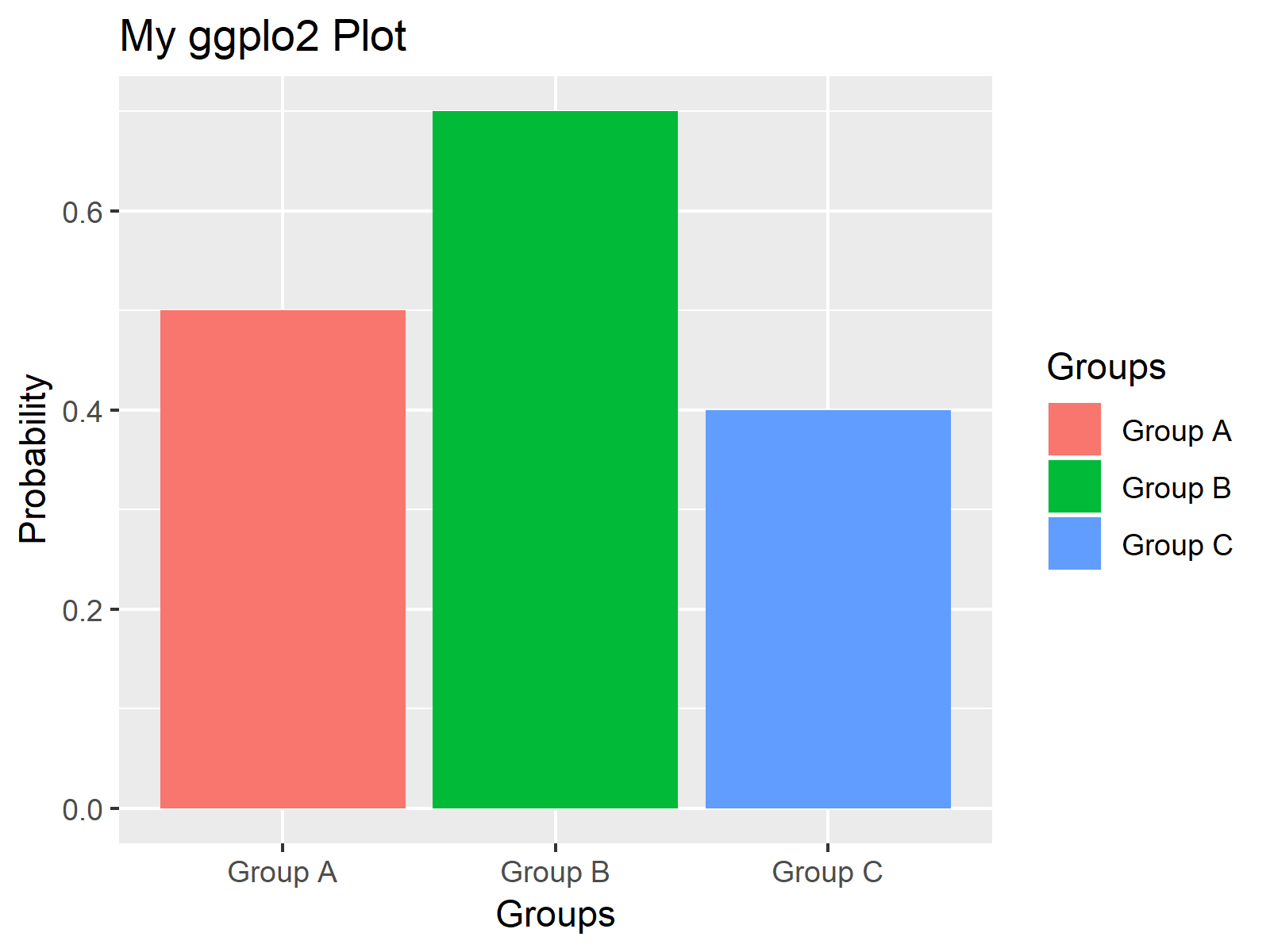Figure 1: ggplot2 Barchart with Default Font Sizes.

Figure 1 illustrates how our example plot looks like. It’s a simple barplot with three bars, each of them representing the probability of a different group.

The font sizes of our barchart are the default sizes. In the following examples, I’ll explain how to change these font sizes with some simple R code.

Let’s move on…

## Example 1: Change Font Size of All Text Elements

In Example 1, I’ll show you how to change all font sizes within your ggplot2 graph with one line of R code. We simply have to specify the element text size within the theme function as shown below:

`my_ggp + theme(text = element_text(size = 20)) # All font sizes`Figure 2: Changing Font Size of All Text Elements.

Figure 2 shows the same graphics as Figure 1, but the font sizes of all text elements are much larger.

Note that you may change the size from 20 to any other value that you want.

In the next examples, I’ll explain how to change only specific text elements of a ggplot2 chart. So keep on reading!

## Example 2: Change Font Size of Axis Text

Example 2 illustrates how to modify the font size of the axis labels. We can either change both axes…

`my_ggp + theme(axis.text = element_text(size = 20)) # Axis text size`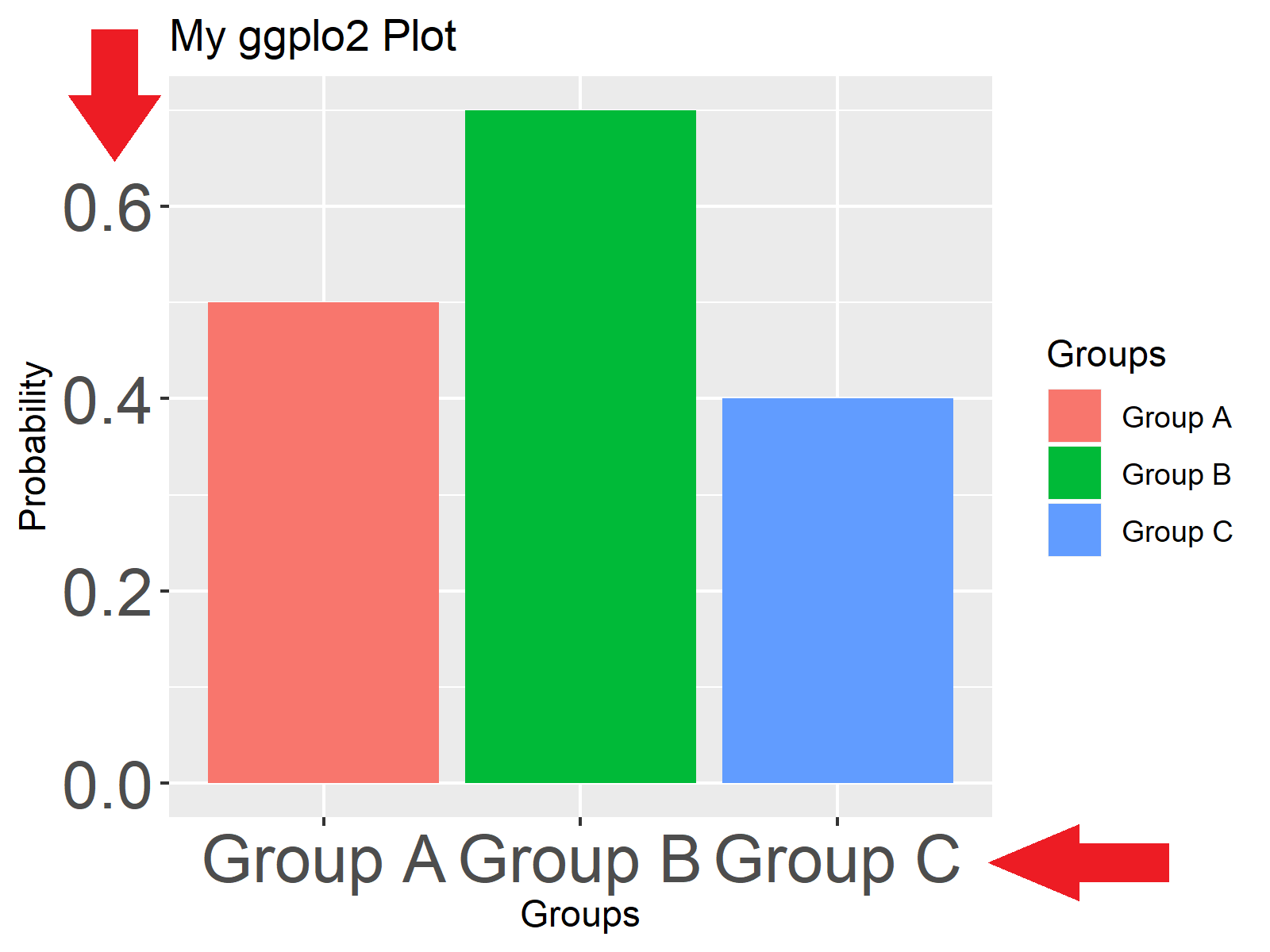Figure 3: Changing Font Size of Axis Text.

…only the x-axis label…

`my_ggp + theme(axis.text.x = element_text(size = 20)) # x-axis text size`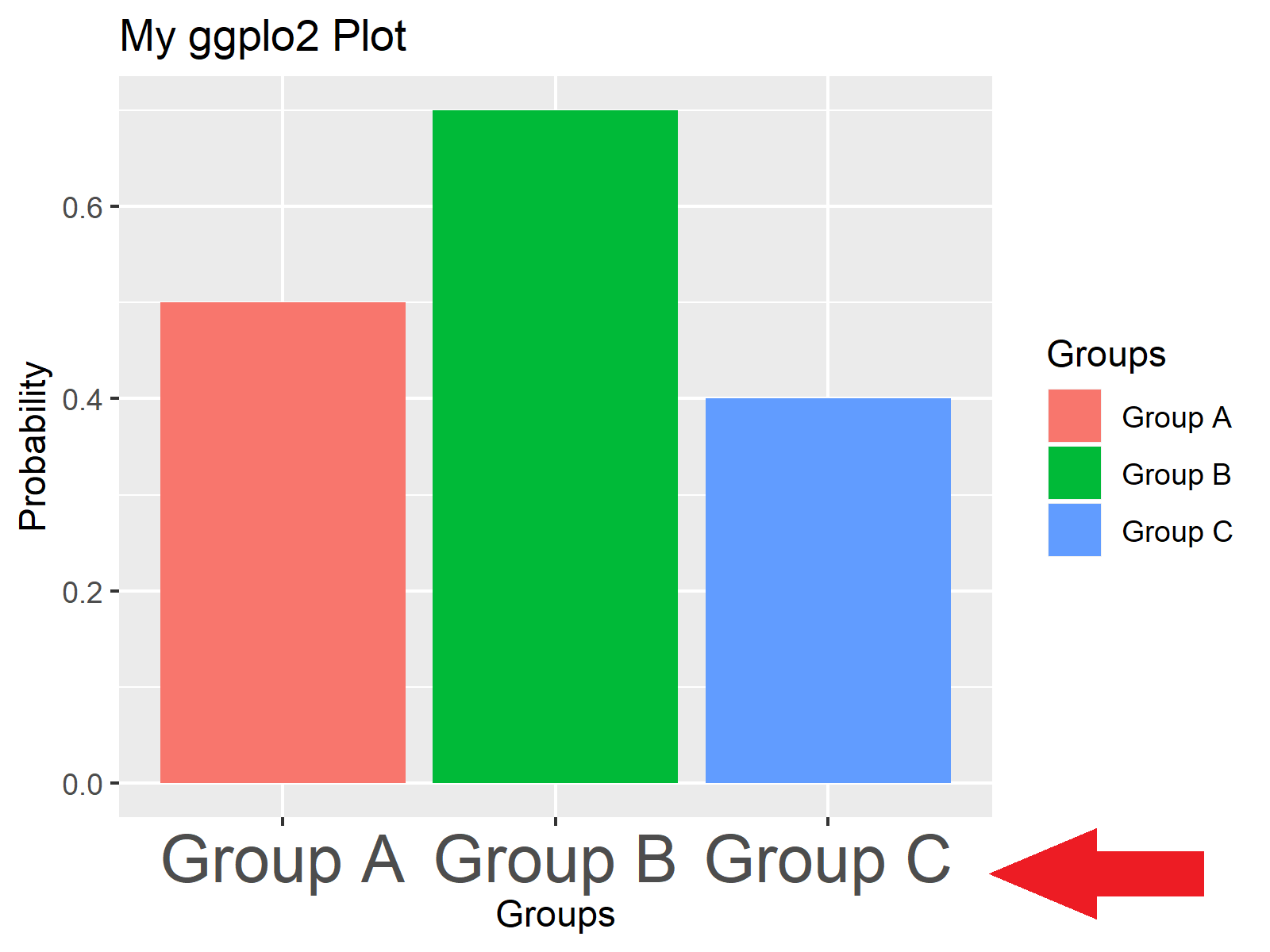Figure 4: Changing Font Size of x-Axis Text.

…or only the y axis:

`my_ggp + theme(axis.text.y = element_text(size = 20)) # y-axis text size`Figure 5: Changing Font Size of y-Axis Text.

## Example 3: Change Font Size of Axis Titles

With the following R syntax, we can change the size of the axis titles of our plot. We can adjust the size of all axis titles…

`my_ggp + theme(axis.title = element_text(size = 20)) # Axis titles`Figure 6: Changing Font Size of Axis Titles.

…only the x-axis title…

`my_ggp + theme(axis.title.x = element_text(size = 20)) # x-axis title`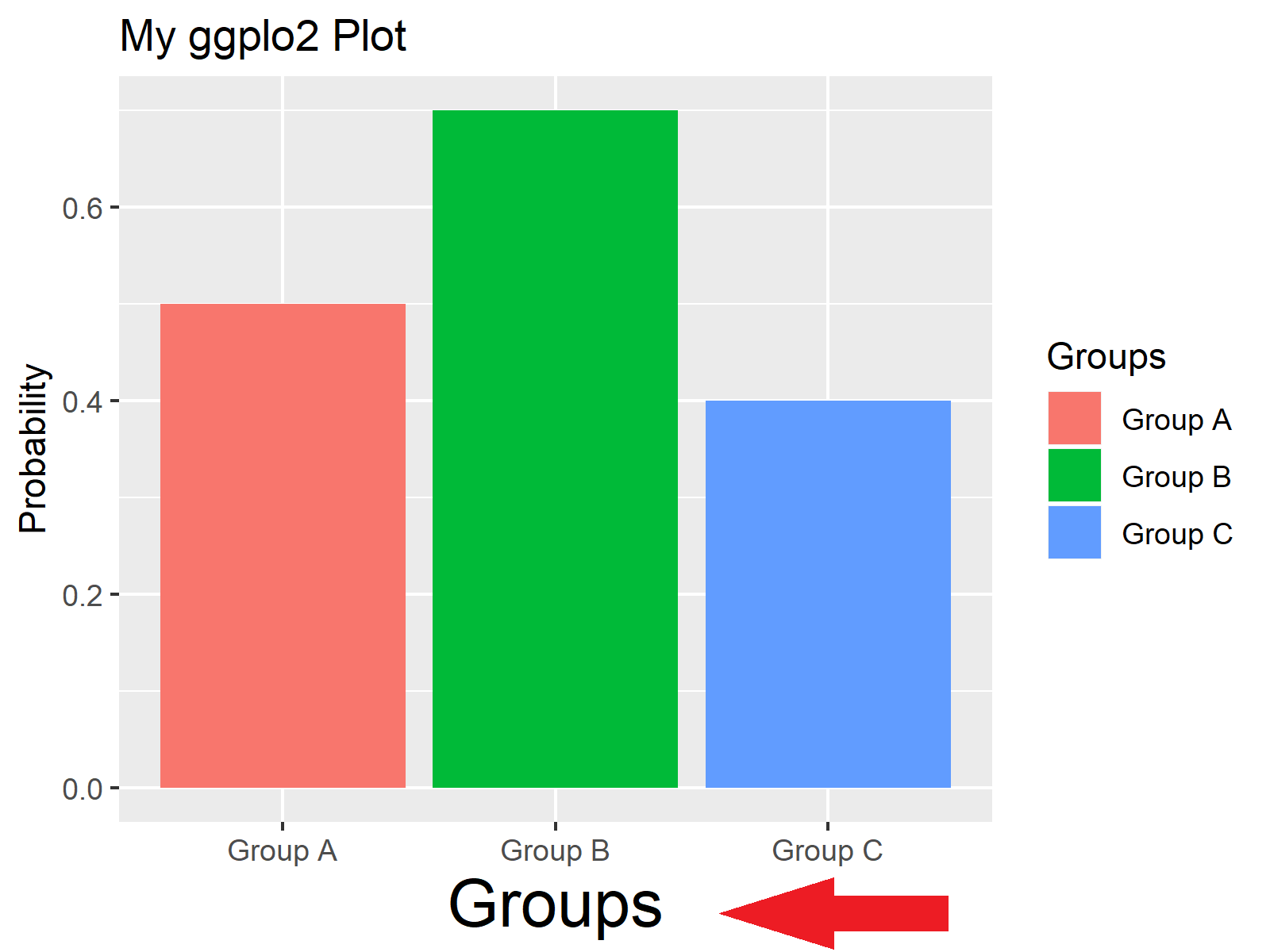Figure 7: Changing Font Size of x-Axis Title.

…or only the y-axis title:

`my_ggp + theme(axis.title.y = element_text(size = 20)) # y-axis title`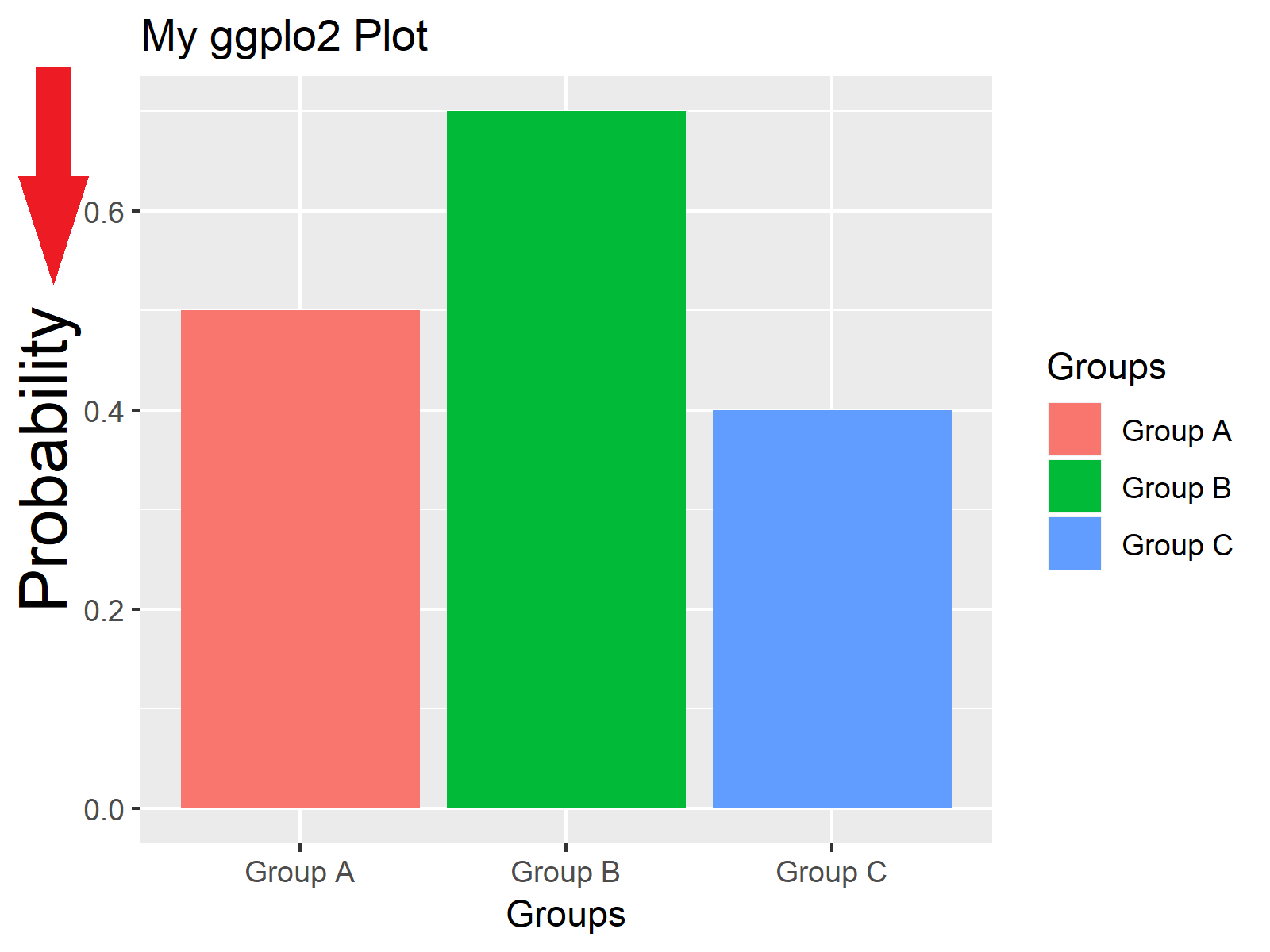Figure 8: Changing Font Size of y-Axis Title.

## Example 4: Change Font Size of Main Title

In this example, you’ll learn how to change the font size of the main title of a ggplot. Have a look at the following R code and the corresponding barchart:

`my_ggp + theme(plot.title = element_text(size = 20)) # Plot title size`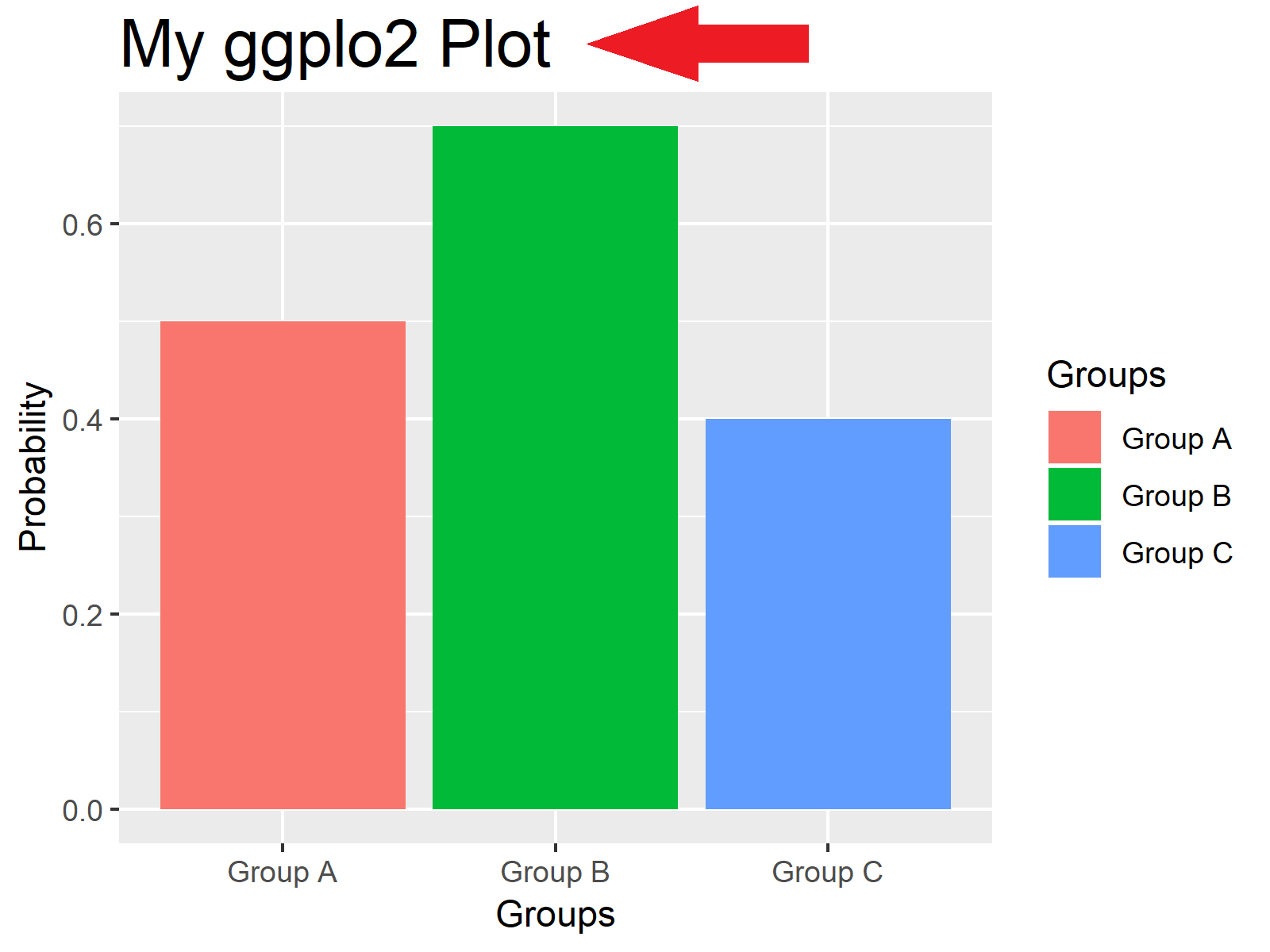Figure 9: Changing Font Size of Main Title.

## Example 5: Change Font Size of Legend

We can also change how large the text elements of a ggplot2 legend are. With the following R syntax, we can increase the text size of the legend text:

`my_ggp + theme(legend.text = element_text(size = 20)) # Legend text`Figure 10: Changing Font Size of Legend Text.

And with the following R code, we can change the size of the legend title:

`my_ggp + theme(legend.title = element_text(size = 20)) # Legend title`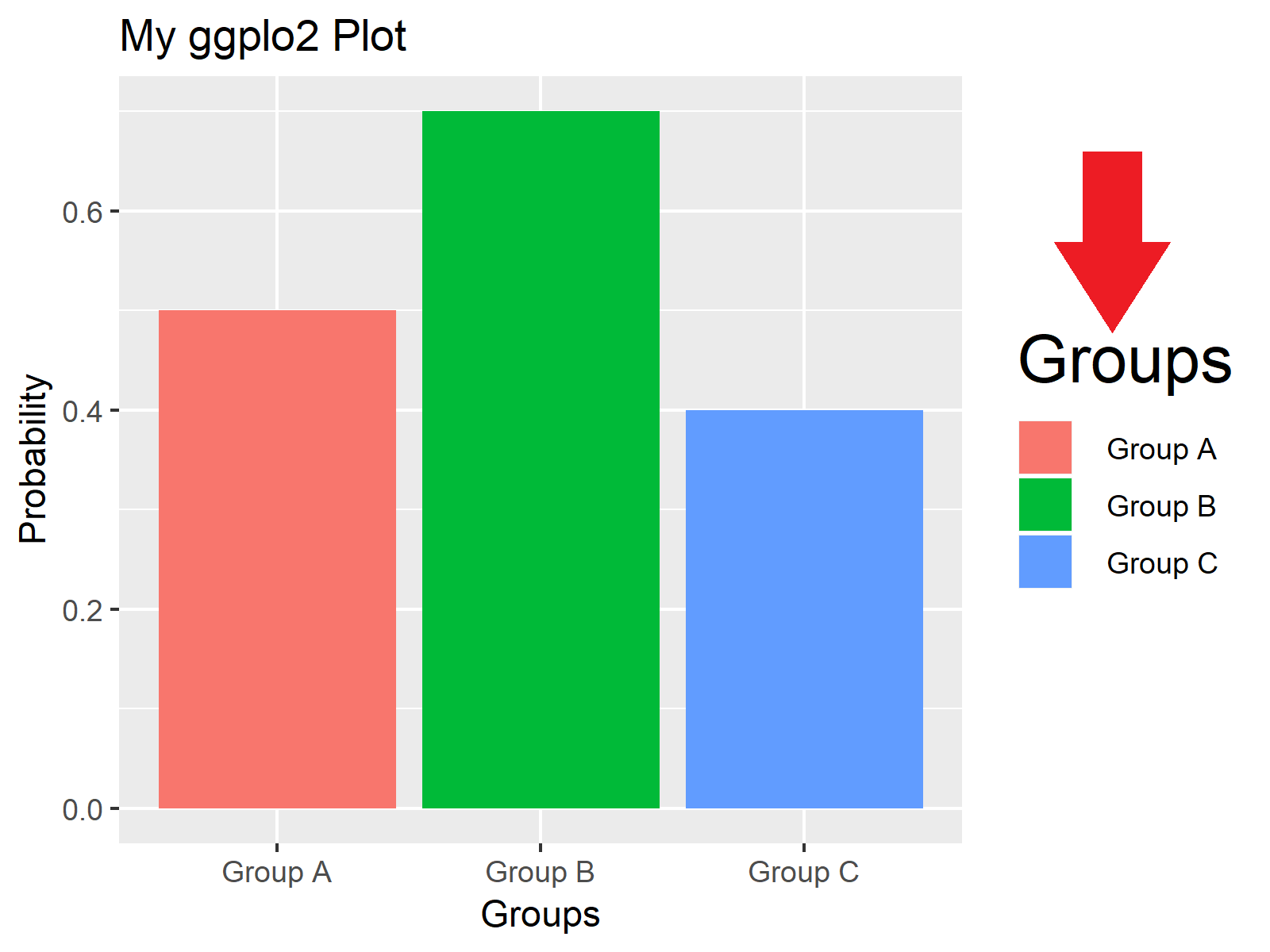Figure 11: Changing Font Size of Legend Title.

## Video & Further Resources

Do you want to learn more about font sizes of ggplot2 plots? Then you may want to have a look at the following video tutorial of my YouTube channel. I illustrate the R codes of this post in the video:

Please accept YouTube cookies to play this video. By accepting you will be accessing content from YouTube, a service provided by an external third party.If you accept this notice, your choice will be saved and the page will refresh.

Furthermore, you might want to read some of the other tutorials of my homepage.

On this page you learned how to increase the font size of too small text elements in R programming. If you have any further questions, please tell me about it in the comments below.

Subscribe to the Statistics Globe Newsletter

•Robert Cline SR
December 14, 2019 8:44 pm

I posted on your youtube channel a question: I am getting an error “font family not found in Windows font database.” Why am I getting this error and what is the solution? Thank you.

•Santosh Kumar
June 21, 2020 7:35 am

Thanks.

• Thank you Santosh! 🙂

•Moses
December 17, 2020 6:33 pm

• Thank you Moses, glad it helped!

•Saar
July 3, 2021 9:49 am

Way to go Joachim !!
I’ve been looking for a clear explanation on this for a long time and this is the best I found
Thanks!

• That’s really great to hear Saar, thanks a lot for the nice comment! 🙂

•Olga
January 30, 2022 5:31 pm

Thanks a lot! How can I rotate the X labels say 45 degrees?

•Tasbiul Islam Nibir
May 31, 2022 1:45 pm

Thank you for your effort. This Greatly Helped me.

• Thank you very much Tasbiul, this is really great to hear!

Regards,
Joachim

•Yingying
August 10, 2022 9:16 am

• Thanks a lot Yingying, this is great to hear! 🙂

Regards,
Joachim

•Ramona C.
September 6, 2022 3:06 pm